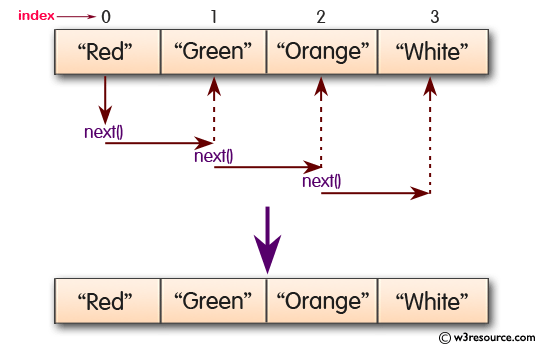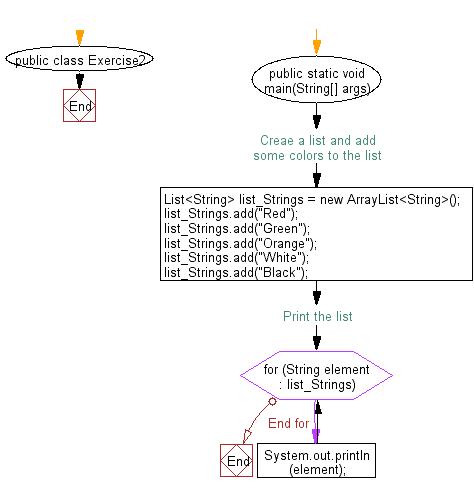﻿ Java: Iterate through all elements in a array list# Java Collection, ArrayList Exercises: Iterate through all elements in a array list

## Java Collection, ArrayList Exercises: Exercise-2 with Solution

Write a Java program to iterate through all elements in a array list.

Pictorial Presentation:Sample Solution:-

Java Code:

``````import java.util.*;
public class Exercise2 {
public static void main(String[] args) {
// Creae a list and add some colors to the list
List<String> list_Strings = new ArrayList<String>();
// Print the list
for (String element : list_Strings) {
System.out.println(element);
}
}
}
``````

Sample Output:

```Red
Green
Orange
White
Black
```

Flowchart:Java Code Editor:

What is the difficulty level of this exercise?

Test your Programming skills with w3resource's quiz.

﻿

## Java: Tips of the Day

countOccurrences

Counts the occurrences of a value in an array.

Use Arrays.stream().filter().count() to count total number of values that equals the specified value.

```public static long countOccurrences(int[] numbers, int value) {
return Arrays.stream(numbers)
.filter(number -> number == value)
.count();
}
```

Ref: https://bit.ly/3kCAgLb